Preliminary results as of Summer 2011
(EPS-HEP and Lepton-Photon conferences)

 The global CKM fit: Inputs and Numerical results The global CKM fit in the large (ρ-bar,η-bar) plane The global CKM fit in the large (ρs-bar,ηs-bar) plane The global CKM fit in the small (ρ-bar,η-bar) plane (zoom) The global CKM fit in the (|Vud|,|Vus|) plane Constraint from BR(B+→τ+ ν) Branching ratio of Bs→μ+ μ- Constraint from decays B→ V γ Constraints on the angle α/ϕ2 from charmless B decays Constraints on the angle γ/ϕ3 from B decays to charm Constraints on |sin(2β+γ)| New physics in neutral-meson mixing

Numerical results:

 The results of the global CKM analysis include: Wolfenstein parameters, UT angles and sides, UTsangle and apex, CKM elements, theory parameters, rare branching fractions (B->lν, B->ll).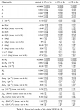Numerical Results

Constraints in the (ρ-bar,η-bar) plane. The red hashed region of the global combination corresponds to 68% CL.
 α, β, γconvention ϕ1, ϕ2, ϕ3convention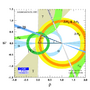eps png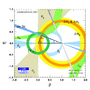eps png
Constraints in the (ρ-bar,η-bar) plane. The |Vub| constraint has been splitted in the two contributions: |Vub| from inclusive and exclusive semileptonic decays (plain dark green) and |Vub| from B+→τ+ ν (hashed green). The red hashed region of the global combination corresponds to 68% CL.
 α, β, γconvention ϕ1, ϕ2, ϕ3convention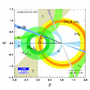eps png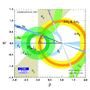eps png

Constraints in the (ρs-bar,ηs-bar) plane. The red hashed region of the global combination corresponds to 68% CL.
 α, β, γconvention ϕ1, ϕ2, ϕ3convention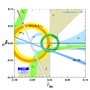eps png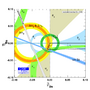eps png

Zoomed constraints in the (ρ-bar,η-bar) plane.The red hashed region of the global combination corresponds to 68% CL.
 α, β, γconvention ϕ1, ϕ2, ϕ3convention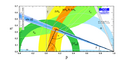eps png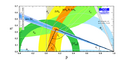eps png
Zoomed constraints in the (ρ-bar,η-bar) plane. The |Vub| constraint has been splitted in the two contributions: |Vub| from inclusive and exclusive semileptonic decays (plain dark green) and |Vub| from B+→τ+ ν (hashed green). The red hashed region of the global combination corresponds to 68% CL.
 α, β, γconvention ϕ1, ϕ2, ϕ3convention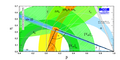eps png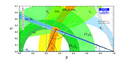eps png
Zoomed constraints in the (ρ-bar,η-bar) plane not including the angle measurements in the global fit.
 α, β, γconvention ϕ1, ϕ2, ϕ3convention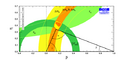eps png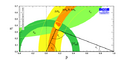eps png
Constraints in the (ρ-bar,η-bar) plane including only the angle measurements.
 α, β, γconvention ϕ1, ϕ2, ϕ3convention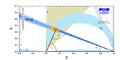eps png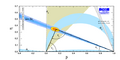eps png
Constraints from CP conserving quantities (|Vub / Vcb|, Δmd, (Δmd and Δms) and B+ →τ+ ν) in the (ρ-bar,η-bar) plane.
 α, β, γconvention ϕ1, ϕ2, ϕ3convention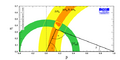eps png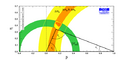eps png
Constraints from CP violating quantities (sin(2β), α, γ and εk) in the (ρ-bar,η-bar) plane.
 α, β, γconvention ϕ1, ϕ2, ϕ3convention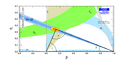eps png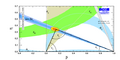eps png
Constraints from "Tree" quantities in the (ρ-bar,η-bar) plane (γ(DK) and α from the isospin analysis with the help of sin2β (charmonium), which gives another tree only γ measurement (the only assumption is that the ΔI=3/2 b-->d EW penguin amplitude is negligible)).
 α, β, γconvention ϕ1, ϕ2, ϕ3convention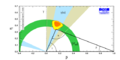eps png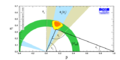eps png
Constraints from "Loop" quantities in the (ρ-bar,η-bar) plane.
 α, β, γconvention ϕ1, ϕ2, ϕ3convention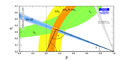eps png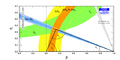eps png
Constraints in the (ρ-bar,η-bar) plane, not including the braching ratio of B+ → τ+ν in the global fit.
 α, β, γconvention ϕ1, ϕ2, ϕ3convention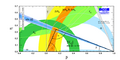eps png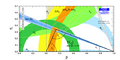eps png
Constraints in the (ρ-bar,η-bar) plane not including the measurement of sin2β in the global fit.
 α, β, γconvention ϕ1, ϕ2, ϕ3convention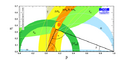eps png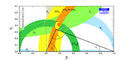eps png

 Constraints in the (|Vud|,|Vus|) plane. The indirect constraints are related to |Vud| and |Vus| through unitarity.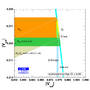eps png

There is a discrepancy in the CKM global fit between the world averages for sin2β and BR(B→τν).
There is a specific correlation between the two quantities in the global fit that is a bit at odds with the direct experimental determination. This is best viewed in the (sin2β,BR(B→τν)) plane, regarding the prediction from the global fit without using these measurements. The cross corresponds to the experimental value with 1 sigma errors.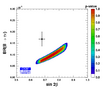eps png

The shape of the correlation can be understood by considering the ratio BR(B→τν)/Δmd, where the decay constant fBd cancels, leaving limited theoretical uncertainties (the ratio depends only on the bag parameter BBd). Thus from the observables BR(B→τν) and Δmd one gets an interesting constraint in the (ρbar,ηbar) plane, which does not match perfectly with the global fit output.
 α, β, γconvention ϕ1, ϕ2, ϕ3convention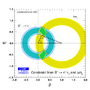eps png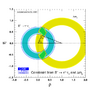eps png
To have a closer look, one can write the full formula for the ratiowhere one explicitly sees that the correlation between BR(B→τν) and the angle β is controlled by the values of BBd, and the angles α and γ. This can be checked explicitly by comparing the above analytical formula with the colored region in the (sin2β,BR(B→τν)) plane. In other words the discrepancy is not driven by the value of semileptonic |Vub|, nor by the decay constant fBd.

To quantify the discrepancy one can compare the indirect fit prediction for BR(B→τν) with the measurement. The deviation here is 2.8 sigmas.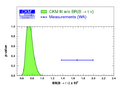eps png

A simpler test is the comparison of the prediction of BBd from the above analytical formula (having only BR(B→τν), Δmd, α, β, γ and |Vud| as inputs, that is an almost completely theory-free determination of BBd) with the current lattice determination BBd = 1.262 +0.083-0.081.

For this test the deviation is 2.8 sigmas, dominated by the error on BR(B→τν), α, γ and BBd.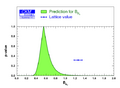eps png

 Prediction for Br(Bs→μ+ μ-)=(3.64+0.18-0.32)x10-9, to be compared with the upper bounds presented at EPS-HEP by LHCb and CMS [<11x10-9 at 95% CL] and CDF [<5.0x10-9 at 90% CL].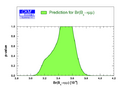eps png

Constraint from B → V γ decays:

 No update See Summer 08 results (here).

Constraints on the angle α/ϕ2 from charmless B decays:

 No update See Moriond 09 results (here).

 Constraints on γ/ϕ3 from world average D(*)K(*) decays (GLW+ADS) and Dalitz analyses (GGSZ) γ[combined] = 68+10-11°, compared to the prediction from the global CKM fit (not including these measurements): γ[fit] = 67.1+4.6-3.7°.eps png Constraint on the ratio of interfering amplitudes rB of the decay B → DK from world average D(*)K(*) decays (GLW+ADS) and Dalitz analyses (GGSZ): rB(DK) = 0.107+0.010-0.010.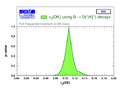eps png Constraint on the ratio of amplitudes rB of the decay B → D*K: rB(D*K) =0.119+0.018-0.019.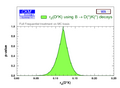eps png Constraint on the ratio of amplitudes rB of the decay B → DK*: rB(DK*) = 0.116+0.045-0.044.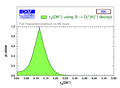eps png Constraint on the strong phase between the interfering amplitudes of the decay B → DK from world average D(*)K(*) decays (GLW+ADS) and Dalitz analyses (GGSZ): δB(DK) = 112+12-13°.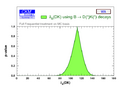eps png Constraint on the strong phase between the interfering amplitudes of the decay B → D*K: δB(D*K) = -55+14-16°.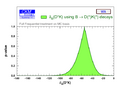eps png Constraint on the strong phase between the interfering amplitudes of the decay B → DK*: δB(DK*) = 117+30-40°.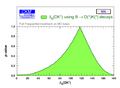eps png

Constraints on |sin(2β+γ)| from the measurement of time-dependent CP asymmetries in D(*) π(ρ); Winter 11 HFAG average. The extraction of the UT-angle combination relies on SU(3) symmetry for the estimates of the suppressed-to-leading amplitude ratios. We use for r(*) the values of the branching fractions for D(*) π(ρ) and Ds(*) π(ρ) as averaged by the PDG group (2011 online update), including Babar 2008 and Belle 2010 results. We use our own average for the ratio fDs/fD equal to 1.185 ± 0.005 ± 0.010. According to arXiv:1109.0460 and in lack of unquenched Nf=2+1 computations, we use a conservative value of 1.1 ± 1.1 for the ratios of the decay constants for D(s)*+π-. We treat the uncertainty on SU(3)-flavour breaking through the method described in Max Baak's thesis (here) with a 10.5% statistic and a 5% systematic uncertainties.
 α, β, γconvention ϕ1, ϕ2, ϕ3conventioneps pngeps png
Translation of this result into γ (using sin(2β) as additional input and choosing among the four solutions to the SM one). γ[GLW+ADS+GGSZ+|sin(2β+γ)|] = (69.1 +9.4-9.9)°.
 α, β, γconvention ϕ1, ϕ2, ϕ3convention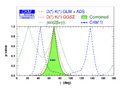eps png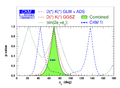eps png
Constraints from |sin(2β+γ)| in the (ρ-bar,η-bar) plane.
 α, β, γconvention ϕ1, ϕ2, ϕ3convention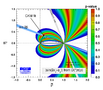eps png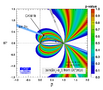eps png
 See the specific studies performed for Lepton Photon 2011 (here).